# Logical Reasoning for Freshers - Set 2

1)   In each of the following questions, choose the correct code form.

In a certain code, MEN is written as ABC and DARK is written as LSTZ. How can READ be written in this code?

a. TCSL
b. TBSL
c. TASL
d. TLSL

Explanation:

 Letter M E N D A R K Code A B C L S T Z
 Letter R E A D Code T B S L

2)   In the following question, choose the correct code form.

In a code language MOBILE is written as ASDFGH, STORK is written as PLSQW. How is MOTORBIKE written in that code?

a. ASLSQDFWH
b. ASLSQDHWH
c. ASLSGHQDW
d. ASLSASFWH

Explanation:

 Letter M O B I L E S T R K Code A S D F G H P L Q W
 Letter M O T O R B I K E Code A S L S Q D F W H

3)   Choose the number which is different from others.

a. 275
b. 183
c. 242
d. 119
e. 369
 Answer  Explanation ANSWER: 242 Explanation: All other numbers are odd numbers, except 242.

4)   Choose the number which is different from others in the group.

a. 48
b. 50
c. 82
d. 170
e. 290
 Answer  Explanation ANSWER: 48 Explanation: All other numbers except 48 are one more than the square of a certain number. Hence, the answer is A.50 = 49 + 182 = 81 + 1170 = 169 + 1290 = 289 + 148 = 49 - 1

1. Pepper Potts, Hulk, Ironman, Hawkeye, Thor, Loki and Black Widow are sitting in a circle.
2. Pepper Potts, Hulk, Ironman, Hawkeye, Loki and Black Widow are sitting at equal distances from each other.
3. Ironman is sitting two places right of Loki, who is sitting one place right of Hawkeye.
4. Pepper Potts forms an angle of 90° from Thor and an angle of 120° from Hulk.
5. Hulk is just opposite Black Widow and is sitting on the left of Thor.

Who is the only person sitting on the immediate left of Black Widow?

a. Loki
b. Hawkeye
c. Thor
d. Pepper Potts
 Answer  Explanation ANSWER: Pepper Potts Explanation: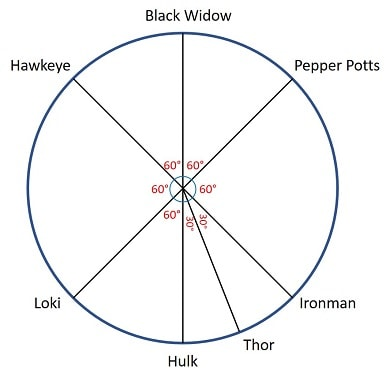Pepper Potts is sitting on the immediate left of Black Widow.

1. Pepper Potts, Hulk, Ironman, Hawkeye, Thor, Loki and Black Widow are sitting in a circle.
2. Pepper Potts, Hulk, Ironman, Hawkeye, Loki and Black Widow are sitting at equal distances from each other.
3. Ironman is sitting two places right of Loki, who is sitting one place right of Hawkeye.
4. Pepper Potts forms an angle of 90° from Thor and an angle of 120° from Hulk.
5. Hulk is just opposite Black Widow and is sitting on the left of Thor.

Thor is sitting between which of the following pair?

a. Ironman and Loki
b. Hulk and Loki
c. Hawkeye and Pepper Potts
d. Hulk and Ironman
 Answer  Explanation ANSWER: Hulk and Ironman Explanation:Thor is sitting between Ironman and Hulk.

7)   In the following question, various terms of an alphabet series are given with one or more missing terms as shown by (?). Choose the missing term out of the given alternatives.

W, P, I, B, ?

a. U
b. V
c. W
d. X
 Answer  Explanation ANSWER: U Explanation: The pattern in the given series is -7i.e. W __-7__ P __-7__ I __-7__ B __-7__ U So, the next term should be U.

8)   In the following question, various terms of an alphabet series are given with one or more missing terms as shown by (?). Choose the missing term out of the given alternatives.

AB, DE, GH, JK, ?

a. LM
b. OP
c. MN
d. PQ
 Answer  Explanation ANSWER: MN Explanation: The pattern in the given series is +2AB __+2 __ DE __+2__ GH __+2__ JK __+2__ MN So, the next term should be MN.

9)   In the question below is given a statement followed by two courses of action numbered I and II. You have to assume everything in the statement to be true and based on the information given in the statement, decide which of the suggested courses of action logically follow(s) for pursuing.

(A) If only I follows
(B) If only II follows
(C) If either I or II follows
(D) If neither I nor II follow
(E) If both I and II follow.

Statement - Incessant rain for the past several days has led to flood in district.

Courses of Action - I. The people residing near potentially dangerous areas should be shifted to a safe place and the Government should install camps to help people.

II. The people residing in other flood prone areas in the state should be made aware about the imminent danger.

III. The government should fling into action with its disaster management plan immediately to control any major loss of life and property.

a. Only I follows
b. Only II follows
c. Only III follows
d. Only I and II follow
e. All follow
 Answer  Explanation ANSWER: All follow Explanation: All the courses given here must be systematically implemented and are equally important to ensure safety of people and prevent further loss to life and property. Hence, all the courses follow.

10)   In the question below is given a statement followed by two courses of action numbered I and II. You have to assume everything in the statement to be true and based on the information given in the statement, decide which of the suggested courses of action logically follow(s) for pursuing.

(A) If only I follows
(B) If only II follows
(C) If either I or II follows
(D) If neither I nor II follow
(E) If both I and II follow.

Statement - Poverty is increasing because the people taking decisions to remove it are not familiar with problems of the poor.

Courses of Action - I. The decision makers should visit and understand problems at village level.

II. Representatives should not be from the poorer sections of the society.

III. A new set of rules and procedures should replace the existing ones.

a. Only I follows
b. Only II follows
c. Either I or II follows
d. All follow
e. Both I and II follow
 Answer  Explanation ANSWER: Only I follows Explanation: The statement indirectly asserts that the decision makers can work effectively to eliminate poverty.The solution to this would be found only if they get to know the basic problems of the poor people through interaction with them. So, here only I follows.

11)   In the following question, a statement/group of statements is given followed by some conclusions. Without resolving anything yourself, choose the conclusion which logically follows from the statement(s).

Statement - Most articles in the shop are expensive.

a. There are no cheap articles available in that shop.
b. Handmade articles in that shop are cheap.
c. There are cheap articles also in that shop.
d. Handmade articles in the shop are expensive.
 Answer  Explanation ANSWER: There are cheap articles also in that shop. Explanation: No explanation is available for this question!

12)   In the following question, a statement/group of statements is given followed by some conclusions. Without resolving anything yourself, choose the conclusion which logically follows from the statement(s).

Statement - All guilty singers were arrested. Narendra and Arvind were among those arrested.

a. All singers are guilty.
b. All arrested people are singers.
c. Narendra and Arvind were not singers.
d. Narendra and Arvind were guilty.
 Answer  Explanation ANSWER: Narendra and Arvind were guilty. Explanation: No explanation is available for this question!

• Raja walked 10 m from A to B in the East.
• Then he turned to the right and walked 3 m.
• Again, he turned to the right and walked 14 m.
How far is Raja from A?

a. 8 m
b. 10 m
c. 12 m
d. 14 m
 Answer  Explanation ANSWER: 10 m Explanation:• Raees walks 10 m towards East and then turns left and walks 12 m.
• Again, he turns right and walks 18 m.
• Finally, he turns to his right and walks 12 m.
How far is Raees from the starting point?

a. 28 m
b. 29 m
c. 30 m
d. 32 m
 Answer  Explanation ANSWER: 28 m Explanation: Required distance = AE = 10 + 18 = 28 m1. P x Q means P is the father of Q
2. P - Q means P is the sister of Q
3. P + Q means P is the mother of Q
4. P ÷ Q means P is the brother of Q

Which of the following represents ‘R is niece of M’?

a. M – J + R – N
b. R – M x T - W
c. M – K x T - R
d. None of these
 Answer  Explanation ANSWER: M – J + R – N Explanation: R is the niece of M means R is the daughter of brother or sister of M i.e. M – J + R – N or M – J x R – N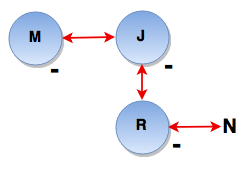1. J + K means J is the son of K
2. J - K means J is the wife of K
3. J x K means J is the brother of K
4. J ÷ K means J is the mother of K
5. J = K means J is the sister of K

What does A + B – C mean?

a. C is the father of A
b. C is the uncle of A
c. C is the son of A
d. C is the brother of A
 Answer  Explanation ANSWER: C is the father of A Explanation: A + B – C means A is the son of B who is the wife of C i.e. C is the father of A.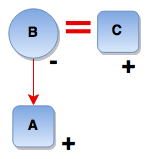17)   A clock is set right at 5 am the clock loses 16 min in 24 hours. What will be the right time when the clock indicates on 3 pm the 8th day?

a. 4 pm
b. 5 pm
c. 6 pm
d. 7 pm
 Answer  Explanation ANSWER: 5 pm Explanation: Time from 5 am On a day to 3 pm on 8th day = 178 hours Now 23 hrs 44 min. of this clock = 24 hours of correct clock 365/15 hrs of this clock = 24 hours of correct clock 178 hrs of this clock = (24 × 15/356 × 89) hrs of correct clock= 180 hrs of correct clock. So, the correct time is 5 pm.

18)   In the question below has a set of three or four statements. Each set of statements is further divided into three segments. Choose the alternative where the third segment in the statement can be logically deduced using both the preceding two, but not just from one of them.

Statement - A. All ugly things are happy. She is ugly. She is happy.
B. All nice things are flat. TVs are flat. TVs are nice things.
C. Potatoes are balls. All balls are fruits. Potatoes are fruits.

a. A
b. B
c. A and B
d. A and C
 Answer  Explanation ANSWER: A and C Explanation: No explanation is available for this question!

19)   In the question given below has a set of three or four statements. Each set of statements is further divided into three segments. Choose the alternative where the third segment in the statement can be logically deduced using both the preceding two, but not just from one of them.

Statement - A. Ravens are white. Ravens are good. All good are white.
B. Insects are faster than eagles. All eagle are hawks. Insects are faster than hawks.
C. No boy is a saint. Peter is a boy. Peter is a saint.

a. A
b. B
c. C
d. None
 Answer  Explanation ANSWER: B Explanation: No explanation is available for this question!

20)   The following question consists of some Problem Figures followed by other figures marked 1, 2, 3, 4 called the Answer Figures.

Find out the correct answer figure that should come nect in the sequence of problem figures.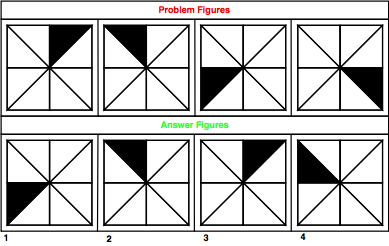a. 1
b. 2
c. 3
d. 4
 Answer  Explanation ANSWER: 4 Explanation: The shaded part of the figure moves in anti-clockwise direction with gaps of 0, 1, 2, 3, 4 and so on. The answer figure should be figure 4.

21)   The following question consists of some Problem Figures followed by other figures marked 1, 2, 3, 4 called the Answer Figures.

Find out the correct answer figure that should come next in the sequence of problem figures.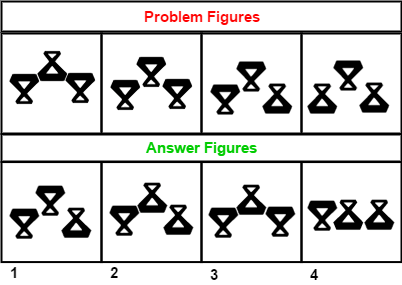a. 1
b. 2
c. 3
d. 4
 Answer  Explanation ANSWER: 1 Explanation: Starting from left to right, the figures are rotated by 180 degree (flipped vertically), one by one in a set order. The answer figure should be figure 1.